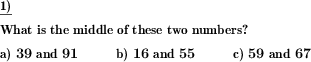Custom math worksheets at your fingertips# Details for problem "Find number in the middle"

Quickname: 3302

Elementary School, Primary School, Junior High School, Middle School.

## Summary

For two given numbers, find the number in the middle, the midpoint.

## Example## Description

Two numbers are given. What is the midpoint between these numbers?

The number of problems, the number range and the number of decimal places of the given number can be chosen.

Please note that the midpoint number may have one more decimal place than the numbers in the problem statement.

Both numbers may be positive or negative, or one may be negative and one positive. This is configurable.

Download free printable worksheets for this math problem here. The worksheet contains the problems only, the solution sheet includes the answers. Just click on the respective link.

•Worksheet 1Solution sheet with answers
•Worksheet 2Solution sheet with answers
•Worksheet 3Solution sheet with answers

If you can not see the solution sheets for download, they may be filtered out by an ad blocker that you may have installed. If this is the case, please allow ads for this page and reload the page. The solution sheets will then reappear.

• Do these sample worksheets do not really fit?
• Do you need more math worksheets, with a different level of difficulty?
• Would you like to combine different problems on a worksheet and adjust them to your needs?
• As a teacher, you can put together your own worksheets using the automatically generated math problems provided.
With a free initial credit, you can start creating your own math worksheets in a few minutes.

You can try it for free! Register here, to create custom worksheets now!

## Customization options for this problem

Parameter
Possible values
Number of problems
1, 2, 3, 4, 5, 6, 7, 8, 9, 10
Number range
20, 30, 40, 50, 100, 200, 500, 1000, 10000, 100000
Decimal places
0, 1, 2, 3
Positive/negative
positive, across origin, positive/negative, negative